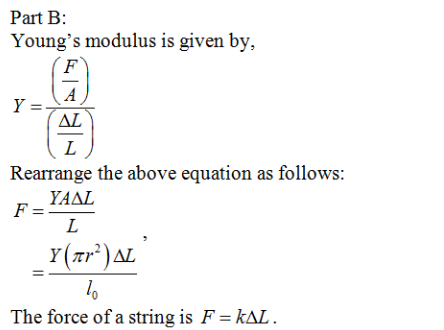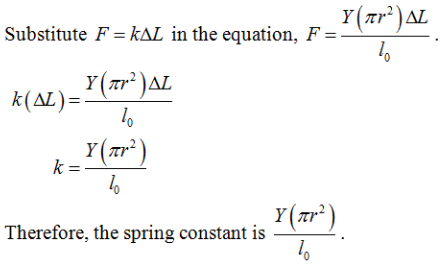# (Part A) When the mass is removed, the length of the cable is found to be l0=4.7m

Consider a cylindrical cable with a hanging weight suspended from it.

(Part A) When the mass is removed, the length of the cable is found to be l0=4.7m. After the mass is added, the length is remeasured and found to be l1=5.25 m. Determine Young’s Modulus Y in N/m2 for the steel cable is the weight has a mass m=35 kg and the cable has a radius r=0.025m.

(Part B) If this cable is pulled down a distance d in m from its equilibrium position it acts like a spring when released. Write an expression determining the spring constant k of this material using the cable-specific variables Y, l1, l0and r provided in part A.